Using the Power Law Model to Evaluate Product Spreading Characteristics on a Rotational Rheometer

The visual and textural perception of a product by a consumer and its behavior during usage can be greatly influenced by its rheological properties. For instance, Newtonian materials will be less responsive to variations in applied stress, whereas very shear thinning materials will show greater dependence. This type of response is critical when considering the ‘spread-ability’ or the ease of spreading.

The thickness of the layer is subsequently reduced as a result of spreading it over a wider surface area (Figure 1). Since the shear rate is defined as the ratio of the velocity and the layer thickness, it is not possible to attribute the process of spreading to a single shear rate. Characterization of the viscosity changes over a range of shear rates will help to assess the spread-ability more successfully (Figure 2).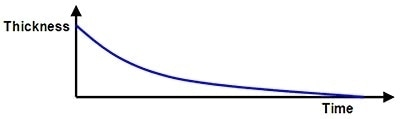Figure 1. Diagram indicating how product layer thickness changes during spreading.

The power law or shear thinning region is the area of interest, as it shows how easily the material structure is deformed upon the application of shear. This region shows linearity with a constant gradient on a log-log plot of viscosity vs. shear rate, but exhibits power law dependence when plotted on a linear scale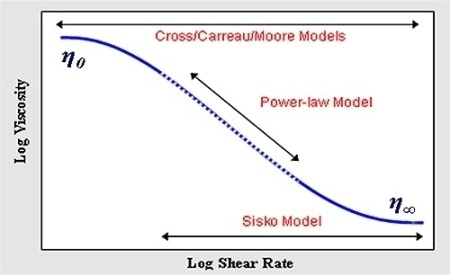Figure 2. Diagram showing an ideal flow curve and the relevant models for describing its shape.

The Power Law or Ostwald de Waele Model can be used to describe this region of the flow curve mathematically as follows: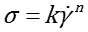Where, k = consistency; n = the power law index; σ = shear rate; and Ý = shear rate.

The unit of consistency is Pasn and its numerical equivalent is the viscosity measured at 1s-1. The range of the power law index is 0 (very shear thinning materials) to 1 (Newtonian materials). The process of spreading should be easier for a material if it requires a lower stress input. If k has a lower value, the viscosity as well as the stress input is eventually lower. If n has a lower value, then the material is shear thinning in nature, which means a smaller increase in the stress with rising shear rate (Figure 3). Therefore, materials possessing low k values and/or low n values should be the easiest to spread.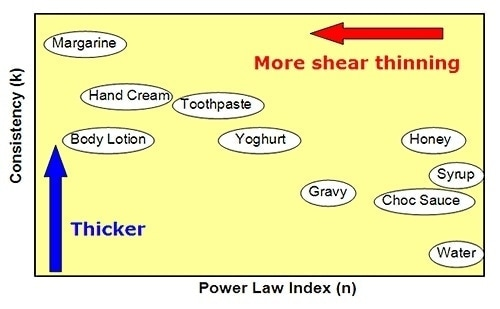Figure 3. Diagram showing how different products may fit on a plot of k versus n.

Experimental

A shear rate ramp test was performed to evaluate the spread-ability of various consumer products, and analyze the resulting curve with a power law model. A Kinexus rotational ,rheometer equipped with a roughened parallel plate measuring system and a Peltier plate cartridge was used to perform rotational rheometer measurements, applying the standard pre-configured sequences in the rSpace software. The use of a standard loading sequence ensured that the samples had undergone a constant and controllable loading protocol. All rheology measurements were carried out at 25°C. A shear rate ramp test was used to generate a flow curve, which was then fitted with a power law model.

Results and Discussion

The viscosity-shear rate curve for various commercial products and their corresponding fitting parameters are presented in Figure 4. Figure 5 depicts the graphical presentation of the fitting parameters. The k value is similar in both toothpaste and hand cream, but hand cream has a much lower n value, making it to spread due to its shear thinning behavior. The k value of syrup and chocolate sauce is much lower, and they do not exhibit shear-thinning behavior and appear sticky and thick during application. The relatively low k and n values make the body lotion easy to spread.Figure 4. Flow curves and model fitting parameters for various samples.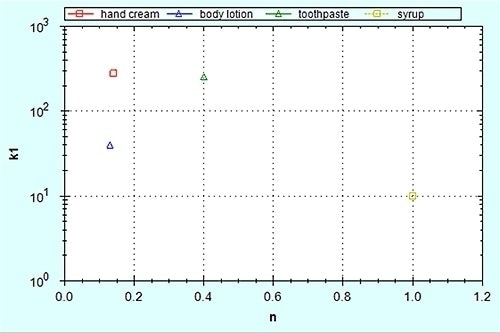Figure 5. Model fitting parameters k and n plotted against one another.

Substituting the k and n values into Equation 1 helps to compare the stress needed to spread the syrup and hand cream at equivalent shear rates quantitatively. When a single shear rate of 1s-1 is considered, which would relate to a thicker layer of a product, then the stress needed to maintain flow at this shear rate is 279 Pa and 10Pa for the hand cream and syrup, respectively (σ = k at 1s-1). The stress needed increases to 10,000 Pa for the syrup and 734 Pa for the hand cream at 1000s-1 shear rate, which would relate to a thinner material layer obtained from the process of spreading. This emphasizes the significance of non-Newtonian behavior in the process of spreading.

Conclusion

This article has discussed the characterization of the spread-ability of a number of commercial products, using a shear rate ramp test with a power law model fit and power law fitting parameters k and n. Low n and k values represent a greater degree of shear thinning and lower viscosity, respectively, facilitating the spreading process.This information has been sourced, reviewed and adapted from materials provided by Malvern Panalytical.

For more information on this source, please visit Malvern Panalytical.

Citations

Please use one of the following formats to cite this article in your essay, paper or report:

• APA

Malvern Panalytical. (2019, September 03). Using the Power Law Model to Evaluate Product Spreading Characteristics on a Rotational Rheometer. AZoM. Retrieved on October 22, 2019 from https://www.azom.com/article.aspx?ArticleID=12759.

• MLA

Malvern Panalytical. "Using the Power Law Model to Evaluate Product Spreading Characteristics on a Rotational Rheometer". AZoM. 22 October 2019. <https://www.azom.com/article.aspx?ArticleID=12759>.

• Chicago

Malvern Panalytical. "Using the Power Law Model to Evaluate Product Spreading Characteristics on a Rotational Rheometer". AZoM. https://www.azom.com/article.aspx?ArticleID=12759. (accessed October 22, 2019).

• Harvard

Malvern Panalytical. 2019. Using the Power Law Model to Evaluate Product Spreading Characteristics on a Rotational Rheometer. AZoM, viewed 22 October 2019, https://www.azom.com/article.aspx?ArticleID=12759.